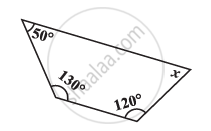Share

# Find the Angle Measure X in the Given Figure - 1 - CBSE Class 8 - Mathematics

ConceptSum of the Measures of the Exterior Angles of a Polygon

#### Question

Find the angle measure x in the given Figure#### Solution

Sum of the measures of all interior angles of a quadrilateral is 360º. Therefore, in the given quadrilateral,

50° + 130° + 120° + x = 360°

300° + x = 360°

x = 60°

Is there an error in this question or solution?

#### APPEARS IN

NCERT Solution for Mathematics Textbook for Class 8 (2018 to Current)